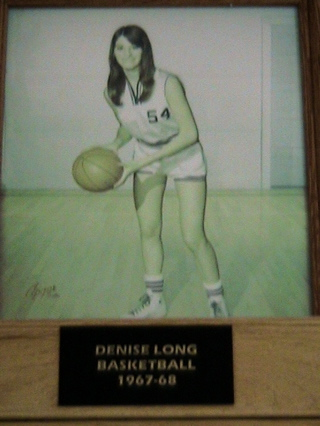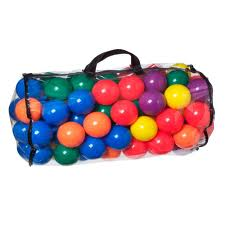Probability

# Pigeonhole Principle: Level 3 ChallengesThere are $51$ people in a room. What is the largest value of $n$ such that the statement "At least $n$ people in this room have birthdays falling in the same month" is always true?

Details and Assumptions

• Assume the probability of each person's birthday is random and independent from one another.In 1969, Denise Long became the first female to be drafted into the NBA. In the previous year, she led the Union-Whitten High School basketball team to the state title, averaging an impressive 62.8 points per game. The most points that she scored in a game that season was an astonishing 111. (This feat was only done once.)

Given that there are 30 games in the season of 1968, what is the smallest possible number of points that she could have scored in her second best game?

Note: She scored a non-negative integer number of points in each game.

A math contest is made up of 52 multiple choice questions each worth either 0 (if wrong or no answer) or 1 (if right). How many students must write the test to be sure that at least 39 have the same final score?In a bag, there are some balls of the same size that are colored by $7$ colors, and for each color the number of balls is $77$. At least how many balls are needed to be picked out to ensure that one can obtain $7$ groups of $7$ balls each such that in each group the balls are monochromatic?

Note: Monochromatic means that all balls in the group are same in color. The balls in different groups can have the same color. For example, if we had 49 balls of the first color, then we are done.

A Professor tells 3 Jokes in his ethics class each year.How large a set of jokes does the professor need in order never to repeat the exact same triple of jokes over a period of 12 years?

×